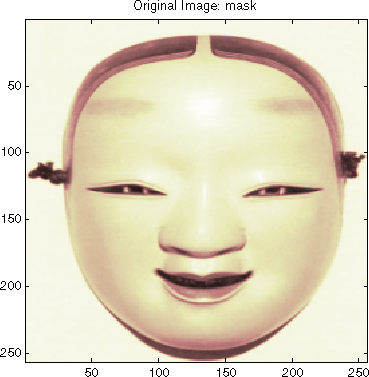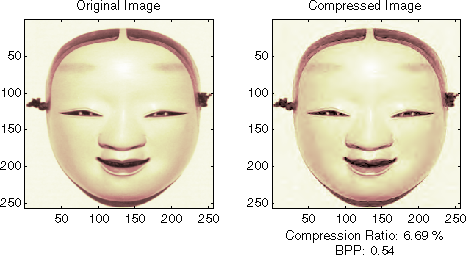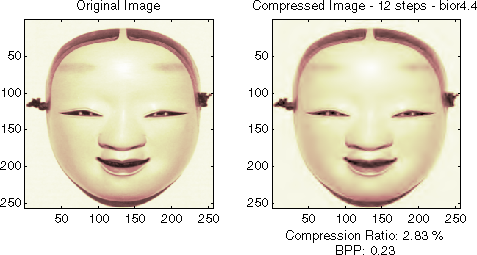## 2-D Wavelet Compression

This section takes you through the features of wavelet 2-D true compression using the Wavelet Toolbox™ software.

For more information on the compression methods see Wavelet Compression for Images in the Wavelet Toolbox User's Guide.

For more information on the main function available when using command-line mode, see the `wcompress` reference pages.

Starting from a given image, the goal of the true compression is to minimize the length of the sequence of bits needed to represent it, while preserving information of acceptable quality. Wavelets contribute to effective solutions for this problem.

The complete chain of compression includes phases of quantization, coding and decoding in addition of the wavelet processing itself.

The purpose of this section is to show how to compress and uncompress a grayscale or truecolor image using various compression methods.

In this section, you'll learn to

• Compress using global thresholding and Huffman encoding

• Uncompress

• Compress using progressive methods

• Handle truecolor images

### 2-D Wavelet Compression Commands

#### Compression by Global Thresholding and Huffman Encoding

```load mask; image(X) axis square; colormap(pink(255)) title('Original Image: mask') ```A synthetic performance of the compression is given by the compression ratio and the Bit-Per-Pixel ratio which are equivalent.

The compression ratio CR means that the compressed image is stored using only CR% of the initial storage size.

The Bit-Per-Pixel ratio BPP gives the number of bits used to store one pixel of the image.

For a grayscale image, the initial BPP is 8 while for a truecolor image the initial BPP is 24 because 8 bits are used to encode each of the three colors (RGB color space).

The challenge of compression methods is to find the best compromise between a weak compression ratio and a good perceptual result.

Let us begin with a simple method cascading global coefficients thresholding and Huffman encoding. We use the default wavelet bior4.4 and the default level which is the maximum possible level (see the `wmaxlev` function) divided by 2.

The desired Bit-Per-Pixel ratio BPP is set to 0.5 and the compressed image will be stored in the file named `'mask.wtc'`.

```meth = 'gbl_mmc_h'; % Method name option = 'c'; % 'c' stands for compression [CR,BPP] = wcompress(option,X,'mask.wtc',meth,'bpp',0.5) CR = 6.6925 BPP = 0.5354 ```

The achieved Bit-Per-Pixel ratio is actually about 0.53 (closed to the desired one) for a compression ratio of 6.7%.

#### Uncompression

Let us uncompress the image retrieved from the file `'mask.wtc'` and compare it to the original image.

```option = 'u'; % 'u' stands for uncompression Xc = wcompress(option,'mask.wtc'); colormap(pink(255)) subplot(1,2,1); image(X); axis square; title('Original Image') subplot(1,2,2); image(Xc); axis square; title('Compressed Image') xlabel({['Compression Ratio: ' num2str(CR,'%1.2f %%')], ... ['BPP: ' num2str(BPP,'%3.2f')]}) ```#### Compression by Progressive Methods

Let us now illustrate the use of progressive methods starting with the well known EZW algorithm using the Haar wavelet. The key parameter is the number of loops. Increasing it, leads to better recovery but worse compression ratio.

```meth = 'ezw'; % Method name wname = 'haar'; % Wavelet name nbloop = 6; % Number of loops [CR,BPP] = wcompress('c',X,'mask.wtc',meth, ... 'maxloop',nbloop,'wname',wname); Xc = wcompress('u','mask.wtc'); colormap(pink(255)) subplot(1,2,1); image(X); axis square; title('Original Image') subplot(1,2,2); image(Xc); axis square; title('Compressed Image - 6 steps') xlabel({['Compression Ratio: ' num2str(CR,'%1.2f %%')], ... ['BPP: ' num2str(BPP,'%3.2f')]}) ```A too small number of steps (here 6) produces a very coarse compressed image. So let us examine a little better result for 9 steps and a satisfactory result for 12 steps.

```[CR,BPP]= wcompress('c',X,'mask.wtc',meth,'maxloop',9, ... 'wname','haar'); Xc = wcompress('u','mask.wtc'); colormap(pink(255)) subplot(1,2,1); image(Xc); axis square; title('Compressed Image - 9 steps') xlabel({['Compression Ratio: ' num2str(CR,'%1.2f %%')],... ['BPP: ' num2str(BPP,'%3.2f')]}) [CR,BPP] = wcompress('c',X,'mask.wtc',meth,'maxloop',12, ... 'wname','haar'); Xc = wcompress('u','mask.wtc'); subplot(1,2,2); image(Xc); axis square; title('Compressed Image - 12 steps') xlabel({['Compression Ratio: ' num2str(CR,'%1.2f %%')],... ['BPP: ' num2str(BPP,'%3.2f')]}) ```As can be seen, the reached BPP ratio is about 0.92 when using 12 steps.

Let us try to improve it by using the wavelet bior4.4 instead of haar and looking at obtained results for steps 12 and 11.

```[CR,BPP] = wcompress('c',X,'mask.wtc','ezw','maxloop',12, ... 'wname','bior4.4'); Xc = wcompress('u','mask.wtc'); colormap(pink(255)) subplot(1,2,1); image(Xc); axis square; title('Compressed Image - 12 steps - bior4.4') xlabel({['Compression Ratio: ' num2str(CR,'%1.2f %%')], ... ['BPP: ' num2str(BPP,'%3.2f')]}) [CR,BPP] = wcompress('c',X,'mask.wtc','ezw','maxloop',11, ... 'wname','bior4.4'); Xc = wcompress('u','mask.wtc'); subplot(1,2,2); image(Xc); axis square; title('Compressed Image - 11 steps - bior4.4') xlabel({['Compression Ratio: ' num2str(CR,'%1.2f %%')], ... ['BPP: ' num2str(BPP,'%3.2f')]}) ```Starting from the eleventh loop, the result can be considered satisfactory. The reached BPP ratio is now about 0.35. It can even be slightly improved by using a more recent method: SPIHT (Set Partitioning In Hierarchical Trees).

```[CR,BPP] = wcompress('c',X,'mask.wtc','spiht','maxloop',12, ... 'wname','bior4.4'); Xc = wcompress('u','mask.wtc'); colormap(pink(255)) subplot(1,2,1); image(X); axis square; title('Original Image') subplot(1,2,2); image(Xc); axis square; title('Compressed Image - 12 steps - bior4.4') xlabel({['Compression Ratio: ' num2str(CR,'%1.2f %%')], ... ['BPP: ' num2str(BPP,'%3.2f')]}) [psnr,mse,maxerr,l2rat] = measerr(X,Xc) delete('mask.wtc') ```The final compression ratio (2.8%) and the Bit-Per-Pixel ratio (0.23) are very satisfactory. Let us recall that the first ratio means that the compressed image is stored using only 2.8% of the initial storage size.

#### Handling Truecolor Images

Finally, let us illustrate how to compress the `wpeppers.jpg` truecolor image. Truecolor images can be compressed along the same scheme as the grayscale images by applying the same strategies to each of the three color components.

The progressive compression method used is SPIHT (Set Partitioning In Hierarchical Trees) and the number of encoding loops is set to 12.

```X = imread('wpeppers.jpg'); [CR,BPP] = wcompress('c',X,'wpeppers.wtc','spiht','maxloop',12); Xc = wcompress('u','wpeppers.wtc'); subplot(1,2,1); image(X); axis square; title('Original Image') subplot(1,2,2); image(Xc); axis square; title('Compressed Image - 12 steps - bior4.4') xlabel({['Compression Ratio: ' num2str(CR,'%1.2f %%')], ... ['BPP: ' num2str(BPP,'%3.2f')]}) delete('wpeppers.wtc') ```The compression ratio (1.65%) and the Bit-Per-Pixel ratio (0.4) are very satisfactory while maintaining a good visual perception.

### 2-D Wavelet Compression using the Wavelet Analyzer App

In this section, we explore the different methods for 2-D true compression, using the Wavelet Analyzer app.

1. Start the True Compression 2-D Tool.

From the MATLAB® prompt, type `waveletAnalyzer`.

The Wavelet Analyzer appears. Click the True Compression 2-D item. The true compression tool for images appears.

At the MATLAB command prompt, type

`load mask`
In the True Compression 2-D tool, select File > Import Image from Workspace. When the Import from Workspace dialog box appears, select the `X` variable. Click OK to import the data. The image appears at the top left of the window together with the gray level histogram just below.3. Perform a Wavelet Decomposition.

Accept the default wavelet bior4.4 and select 4 from the Level menu which is the maximum possible level divided by 2 and then click the Decompose button. After a pause for computation, the tool displays the wavelet approximation and details coefficients of the decomposition for the three directions, together with the histogram of the original coefficients.The peak of the bin containing zero illustrates the capability of wavelets to concentrate the image energy into a few nonzero coefficients.

4. Try a simple method.

Begin with a simple method cascading global coefficients thresholding and Huffman encoding.

Choose the GBL_MMC_H option from the menu Compression method located at the top right of the Compression Parameters frame. For more information on the compression methods, see Wavelet Compression for Images in the Wavelet Toolbox User's Guide.

Set the desired Bit-Per-Pixel ratio to 0.5.Values of the other parameters are automatically updated. Note that these values are only approximations of the true performances since the quantization step cannot be exactly predicted without performing it. Click the Compress button.Synthetic performance is given by the compression ratio and the computed Bit-Per-Pixel (BPP). This last one is actually about 0.53 (close to the desired one 0.5) for a compression ratio of 6.7%.

The compressed image, at the bottom left, can be compared with the original image.

The result is satisfactory but a better compromise between compression ratio and visual quality can be obtained using more sophisticated true compression which combines the thresholding and quantization steps.

5. Compress using a first progressive method: EZW.

Let us now illustrate the use of progressive methods starting with the well known EZW algorithm. Let us start by selecting the wavelet haar from the Wavelet menu and select 8 from the Level menu. Then click the Decompose button.

Choose the EZW option from the menu Compression method. The key parameter is the number of loops: increasing it leads to a better recovery but worse compression ratio. From the Nb. Encoding Loops menu, set the number of encoding loops to 6, which is a small value. Click the Compress button.6. Refine the result by increasing the number of loops.

Too few steps produce a very coarse compressed image. So let us examine a little better result for 9 steps. Set the number of encoding loops to 9 and click the Compress button.As can be seen, the result is better but not satisfactory, both by visual inspection and by calculating the Peak Signal to Noise Ratio (PSNR) which is less than 30.Now try a large enough number of steps to get a satisfactory result. Set the number of encoding loops to 12 and click the Compress button.The result is now acceptable. But for 12 steps, we attain a Bit-Per-Pixel ratio about 0.92.

7. Get better compression performance by changing the wavelet and selecting the best adapted number of loops.

Let us try to improve the compression performance by changing the wavelet: select bior4.4 instead of haar and then click the Decompose button.

In order to select the number of loops, the Wavelet Analyzer app tool allows you to examine the successive results obtained by this kind of stepwise procedure. Set the number of encoding loops at a large value, for example 13, and click the Show Compression Steps button. Moreover you could execute the procedure stepwise by clicking the Stepwise button.Then, click the Compress button. Thirteen progressively more finely compressed images appear, and you can then select visually a convenient value for the number of loops. A satisfactory result seems to be obtained after 11 loops. So, you can set the number of encoding loops to 11 and click the Compress button.

The reached BPP ratio is now about 0.35 which is commonly considered a very satisfactory result. Nevertheless, it can be slightly improved by using a more recent method SPIHT (Set Partitioning In Hierarchical Trees).

8. Obtain a final compressed image by using a third method.

Choose the SPIHT option from the menu Compression method, set the number of encoding loops to 12, and click the Compress button.The final compression ratio and the Bit-Per-Pixel ratio are very satisfactory: 2.8% and 0.22. Let us recall that the first ratio means that the compressed image is stored using only 2.8% of the initial storage size.

9. Handle truecolor images.

Finally, let us illustrate how to compress truecolor images. The truecolor images can be compressed along the same scheme by applying the same strategies to each of the three-color components.

From the File menu, choose the Load Image option and select the Matlab Supported Formats item.

When the Load Image dialog box appears, select the MAT-file `wpeppers.jpg` which should reside in the MATLAB folder `toolbox/wavelet/wavelet`.

Click the OK button. A window appears asking you if you want to consider the loaded image as a truecolor one. Click the Yes button. Accept the defaults for wavelet and decomposition level menus and then click the Decompose button.

Then, accept the default compression method SPIHT and set the number of encoding loops to 12. Click the Compress button.

The compression ratio and Bit-Per-Pixel (BPP) ratio are very satisfactory: 1.65% and 0.4 together with a very good perceptual result.10. Inspect the wavelet tree.

For both grayscale and truecolor images, more insight on the multiresolution structure of the compressed image can be retrieved by clicking the Inspect Wavelet Trees button and then on the various active menus available from the displayed tree.### Importing and Exporting from the Wavelet Analyzer App

You can save the compressed image to disk in the current folder with a name of your choice.

The Wavelet Toolbox compression tools can create a file using either one of the Matlab Supported Format types or a specific format which can be recognized by the extension of the file: wtc (Wavelet Toolbox Compressed).

To save the above compressed image, use the menu option File > Save Compressed Image > Wavelet Toolbox Compressed Image. A dialog box appears that lets you specify a folder and filename for storing the image. Of course, the use of the wtc format requires you to uncompress the stored image using the Wavelet Toolbox true compression tools.

## SupportGet trial now